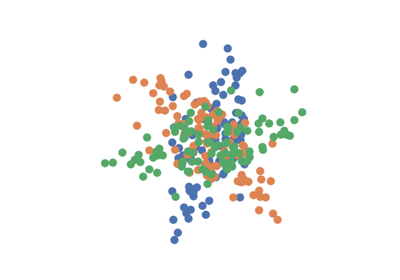# MANOVA¶

class hyppo.ksample.MANOVA

Multivariate analysis of variance (MANOVA) test statistic and p-value.

MANOVA is the current standard for multivariate k-sample testing.

Notes

The test statistic is formulated as below 1:

In MANOVA, we are testing if the mean vectors of each of the k-samples are the same. Define $$\{ {x_1}_i \stackrel{iid}{\sim} F_{X_1},\ i = 1, ..., n_1 \}$$, $$\{ {x_2}_j \stackrel{iid}{\sim} F_{X_2},\ j = 1, ..., n_2 \}$$, ... as k groups of samples deriving from different a multivariate Gaussian distribution with the same dimensionality and same covariance matrix. That is, the null and alternate hypotheses are,

$\begin{split}H_0 &: \mu_1 = \mu_2 = \cdots = \mu_k, \\ H_A &: \exists \ j \neq j' \text{ s.t. } \mu_j \neq \mu_{j'}\end{split}$

Let $$\bar{x}_{i \cdot}$$ refer to the columnwise means of $$x_i$$; that is, $$\bar{x}_{i \cdot} = (1/n_i) \sum_{j=1}^{n_i} x_{ij}$$. The pooled sample covariance of each group, $$W$$, is

$W = \sum_{i=1}^k \sum_{j=1}^{n_i} (x_{ij} - \bar{x}_{i\cdot} (x_{ij} - \bar{x}_{i\cdot})^T$

Next, define $$B$$ as the sample covariance matrix of the means. If $$n = \sum_{i=1}^k n_i$$ and the grand mean is $$\bar{x}_{\cdot \cdot} = (1/n) \sum_{i=1}^k \sum_{j=1}^{n} x_{ij}$$,

$B = \sum_{i=1}^k n_i (\bar{x}_{i \cdot} - \bar{x}_{\cdot \cdot}) (\bar{x}_{i \cdot} - \bar{x}_{\cdot \cdot})^T$

Some of the most common statistics used when performing MANOVA include the Wilks' Lambda, the Lawley-Hotelling trace, Roy's greatest root, and Pillai-Bartlett trace (PBT) 2 3 (PBT was chosen to be the best of these as it is the most conservative 4) and 5 has shown that there are minimal differences in statistical power among these statistics. Let $$\lambda_1, \lambda_2, \ldots, \lambda_s$$ refer to the eigenvalues of $$W^{-1} B$$. Here $$s = \min(\nu_{B}, p)$$ is the minimum between the degrees of freedom of $$B$$, $$\nu_{B}$$ and $$p$$. So, the PBT MANOVA test statistic can be written as,

$\mathrm{MANOVA}_{n_1, \ldots, n_k} (x, y) = \sum_{i=1}^s \frac{\lambda_i}{1 + \lambda_i} = \mathrm{tr} (B (B + W)^{-1})$

The p-value analytically by using the F statitic. In the case of PBT, given $$m = (|p - \nu_{B}| - 1) / 2$$ and $$r = (\nu_{W} - p - 1) / 2$$, this is:

$F_{s(2m + s + 1), s(2r + s + 1)} = \frac{(2r + s + 1) \mathrm{MANOVA}_{n_1, n_2} (x, y)}{(2m + s + 1) (s - \mathrm{MANOVA}_{n_1, n_2} (x, y))}$

References

1

Sambit Panda, Cencheng Shen, Ronan Perry, Jelle Zorn, Antoine Lutz, Carey E. Priebe, and Joshua T. Vogelstein. Nonpar MANOVA via Independence Testing. arXiv:1910.08883 [cs, stat], April 2021. arXiv:1910.08883.

2

M. S. Bartlett. A note on tests of significance in multivariate analysis. Mathematical Proceedings of the Cambridge Philosophical Society, 35(2):180–185, April 1939. doi:10.1017/S0305004100020880.

3

C. Radhakrishna Rao. Tests of Significance in Multivariate Analysis. Biometrika, 35(1/2):58–79, 1948. doi:10.2307/2332629.

4

Russell Warne. A Primer on Multivariate Analysis of Variance (MANOVA) for Behavioral Scientists. Practical Assessment, Research, and Evaluation, November 2019. doi:10.7275/sm63-7h70.

5

Brian S. Everitt. A Monte Carlo Investigation of the Robustness of Hotelling's One- and Two-Sample T2 Tests. Journal of the American Statistical Association, 74(365):48–51, 1979. doi:10.2307/2286719.

Methods Summary

 MANOVA.statistic(*args) Calulates the MANOVA test statistic. MANOVA.test(*args) Calculates the MANOVA test statistic and p-value.

MANOVA.statistic(*args)

Calulates the MANOVA test statistic.

Parameters

*args (ndarray) -- Variable length input data matrices. All inputs must have the same number of dimensions. That is, the shapes must be (n, p) and (m, p), ... where n, m, ... are the number of samples and p is the number of dimensions.

Returns

stat (float) -- The computed MANOVA statistic.

MANOVA.test(*args)

Calculates the MANOVA test statistic and p-value.

Parameters

*args (ndarray) -- Variable length input data matrices. All inputs must have the same number of dimensions. That is, the shapes must be (n, p) and (m, p), ... where n, m, ... are the number of samples and p is the number of dimensions.

Returns

Examples

>>> import numpy as np
>>> from hyppo.ksample import MANOVA
>>> x = np.arange(7)
>>> y = x
>>> stat, pvalue = MANOVA().test(x, y)
>>> '%.3f, %.1f' % (stat, pvalue)
'0.000, 1.0'


## Examples using hyppo.ksample.MANOVA¶